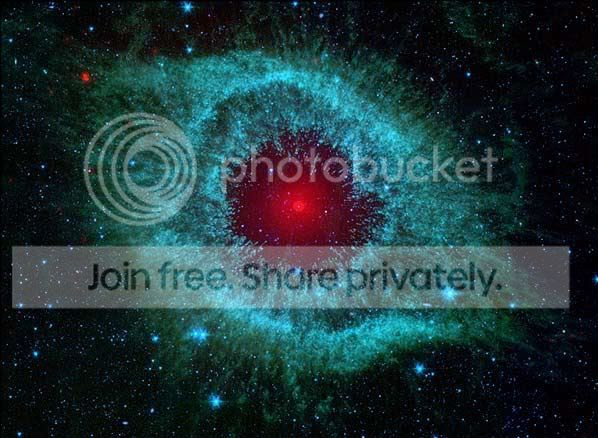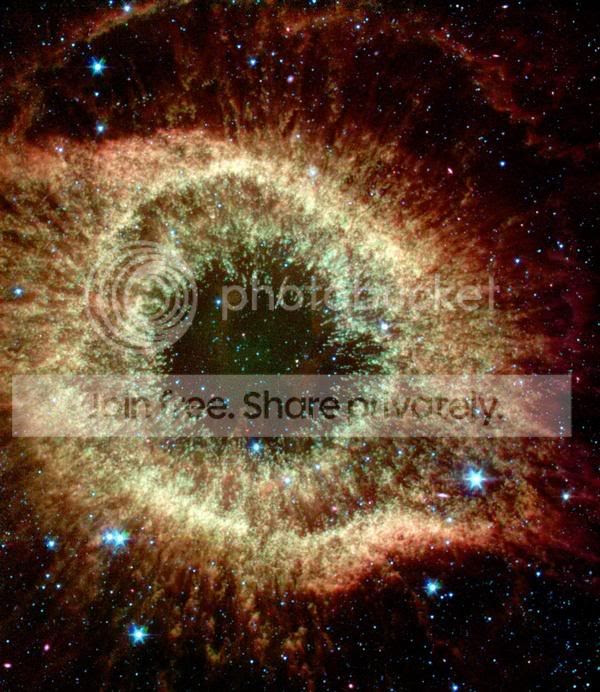### Helix Nebula NGC 7293

A golden rectangle is a rectangle whose side lengths are in the golden ratio, one-to-phi, that is, approximately 1:1.618. A distinctive feature of this shape is that when a square section is removed, the remainder is another golden rectangle, that is, with the same proportions as the first. Square removal can be repeated infinitely, which leads to an approximation of the golden or Fibonacci spiral.

Fibonacci numbers (0,1,1,2,3,5,8,13,21,34...) are a sequence of numbers named after Leonardo of Pisa, known as Fibonacci. The first number of the sequence is 0, the second number is 1, and each subsequent number is equal to the sum of the previous two numbers of the sequence itself.

The Helix Nebula (also known as NGC 7293) is a planetary nebula about 650 light-years away in the constellation Aquarius. It is one of the closest planetary nebulae to Earth.There is a point from which the Golden Spiral appears to emanate, This point is called the Eye of God.